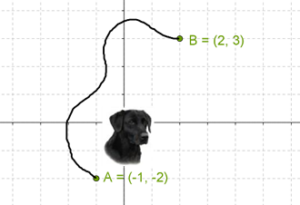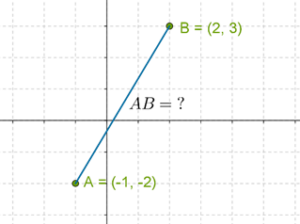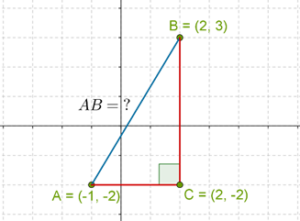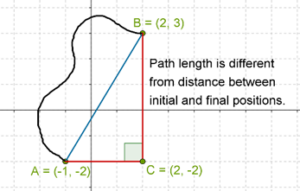In the verge of coronavirus pandemic, we are providing FREE access to our entire Online Curriculum to ensure Learning Doesn't STOP!

# Straight Line Distance

Go back to  'Coordinate-Geometry'

Suppose that Bono walks from point A (whose coordinates are $$\left( { - 1,\; - 2} \right)$$ to point B (whose coordinates are $$\left( {2,\;3} \right)$$, along some random path:What is the distance between Bonos initial and final positions? That is, what is the length AB? Note that the length AB will depend only on the positions (coordinates) of A and B, and not on the particular path Bono took while walking from A to B. Technically speaking, the length AB is said to be the straight line distance between the points A and B, and it is the shortest distance Bono will have to walk if they want to get from point A to point B. We will refer to the straight line distance between A and B as simply the distance between A and B.The question is: given the coordinates of A and B, how do we determine the distance between them? The answer to this question is provided by the distance formula, which is nothing but a simple application of the Pythagoras Theorem, and yet, it is one of the most fundamental results of coordinate geometry.

To determine AB, we complete the right triangle ABC, as shown below. C is the point with the same y coordinate as A and the same x coordinate as B:In this right triangle, AB is the hypotenuse, and so it can be calculated using the Pythagoras Theorem, if we know the values of AC and BC. Consider AC for a moment. It is parallel to the x axis. This means that its length will simply be the difference between the x coordinate of the rightmost point on it, which is C, and the x coordinate of the leftmost point on it, which is A. Thus,

$AC = {x_C} - {x_A} = \left( 2 \right) - \left( { - 1} \right) = 3$

Now, consider BC. It is parallel to the y axis, which means that its length will be the difference between the y coordinate of the topmost point on it, which is B, and the y coordinate of the lowermost point on it, which is C. Thus,

$BC = {y_B} - {y_C} = \left( 3 \right) - \left( { - 2} \right) = 5$

Now that we have AC and BC, AB can be calculated using the Pythagoras Theorem:

$\begin{array}{l}AB = \sqrt {A{C^2} + B{C^2}} \\ \qquad = \sqrt {{3^2} + {5^2}} \\ \qquad = \sqrt {34} \;{\rm{units}}\end{array}$

Once again, note that the actual distance covered by Bono (along the random, wiggly path) will be different (greater) than the distance between his initial and final positions:This distinction will become extremely important later on in your studies.

Coordinate Geometry
Grade 9 | Questions Set 1
Coordinate Geometry
Coordinate Geometry
Grade 10 | Questions Set 1
Coordinate Geometry